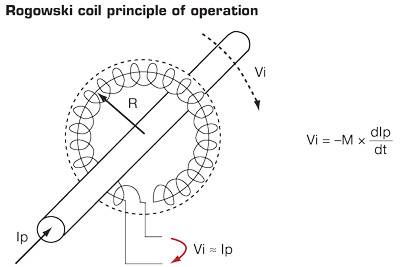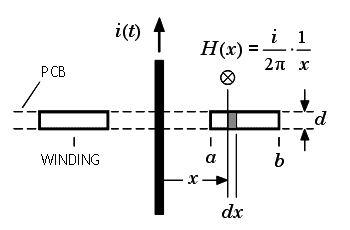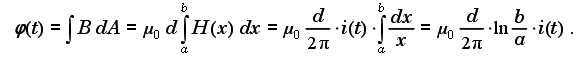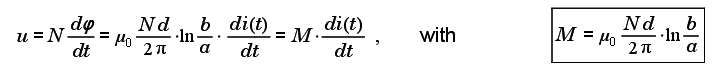# How to calculate mutual inductance of a rectangular loop

I am planning to build a rogowski coil but not the conventional one, which is a circular loop.
Below is the example of a rogowski coil so you have the big picture of my question.In the picture, the current conducting wire (with current Ip) is encircled by a big circular loop rogowski coil. The cross sectional area of the winding itself can be a circle, rectangle, or oval.
For this variations of cross sectional area, I know how to calculate the mutual inductance.

However, what if the shape of the big loop is rectangular? How to calculate the mutual inductance?

I hope you all can help me figure this out.

Hesch
Gold Member
However, what if the shape of the big loop is rectangular? How to calculate the mutual inductance?

Think of Amperes law, which in this case could be formulated:

circulation H⋅ds = Ip

( B = μ0*H )

What happens if you choose a rectangle as integration path instead of a circle?

Think of Amperes law, which in this case could be formulated:

circulation H⋅ds = Ip

( B = μ0*H )

What happens if you choose a rectangle as integration path instead of a circle?

Let's see. Suppose I is 1kA.
So:

circulation B⋅ds = Ip0

circulation B⋅ds = 1000 A * 4π*10-7 Vs/Am = 4π*10-4

So then, how is the B ?

Last edited:
Hesch
Gold Member
Forget B for a moment. Let's look at H when Ip = 1000A and radius for the "big loop" = R, according to your drawing in #1:

circulation H⋅ds = 1000A

Now you choose a circulation path through the "small loops", giving:

H = 1000A / (2πR). Here 2πR is the length of the rogowski coil = L ⇒ H = 1000A / L.

Now you bend the the coil into a rectangle, and you choose a circulation path following this rectangular shape. The length of the rogowski coil is still the same = L, you have just bended it. So now Hmean = 1000A / L. This means that

Hmean = H ⇒
Bmean = B ⇒
Vicircle = dψv/dt = Virect. ( Ψv = flux * turns )

According to the definition: Vi = -M × dIp/dt ⇒
Mrect = Mcircle

Last edited:
Forget B for a moment. Let's look at H when Ip = 1000A and radius for the "big loop" = R, according to your drawing in #1:

circulation H⋅ds = 1000A

Now you choose a circulation path through the "small loops", giving:

H = 1000A / (2πR). Here 2πR is the length of the rogowski coil = L ⇒ H = 1000A / L.

Now you bend the the coil into a rectangle, and you choose a circulation path following this rectangular shape. The length of the rogowski coil is still the same = L, you have just bended it. So now Hmean = 1000A / L. This means that

Hmean = H ⇒
Bmean = B ⇒
Vicircle = dψv/dt = Virect. ( Ψv = flux * turns )

According to the definition: Vi = -M × dIp/dt ⇒
Mrect = Mcircle

Okay, let's say Mrect = Mcircle

And suppose the small loop is rectangular as well.To calculate M:So:The integration is easy since the distance x from the current wire to the coil is all the same because of circular symmetry of the big loop. However, if the big loop is rectangular, the distance x will be different for each small loop. Also, it will make a and b change all the time as well. So, how to calculate this?

Last edited:
Hesch
Gold Member
The integration is easy since the distance x from the current wire to the coil is all the same because of circular symmetry of the big loop. However, if the big loop is rectangular, the distance x will be different for each small loop. Also, it will make a and b change all the time as well. So, how to calculate this?

You may calculate it using Biot-Savart law, but that is comprehensive.

I think that the idea is to look at: circulation H⋅ds = 1000A. This equation is valid no matter what circulation path you choose. Now say that the distance between two windings in the rogowski coil is very small ( ds ), and the length of the big loop is constant, then the mean value of H will be the same as for rectangular/circular shape of the big loop.

Hmean = constant → Bmean = constant → Ψv = constant → Vi = constant → M = constant. ( Ψv = flux * ( number of windings ) ).

So the idea is to regard the rectangular big loop as a circular loop as you will get the same result in calculations under the conditions:

Same length of the big loop, same number of turns in the rogowski coil, same cross section area of the rogowski coil, the rogowski coil is completely surrounding the conductor (closed loop).

Last edited:
You may calculate it using Biot-Savart law, but that is comprehensive.

I think that the idea is to look at: circulation H⋅ds = 1000A. This equation is valid no matter what circulation path you choose. Now say that the distance between two windings in the rogowski coil is very small ( ds ), and the length of the big loop is constant, then the mean value of H will be the same as for rectangular/circular shape of the big loop.

Hmean = constant → Bmean = constant → Ψv = constant → Vi = constant → M = constant. ( Ψv = flux * ( number of windings ) ).

So the idea is to regard the rectangular big loop as a circular loop as you will get the same result in calculations under the conditions:

Same length of the big loop, same number of turns in the rogowski coil, same cross section area of the rogowski coil, the rogowski coil is completely surrounding the conductor (closed loop).

Okay then. I will try to make both coils and compare the result. Thanks a lot.